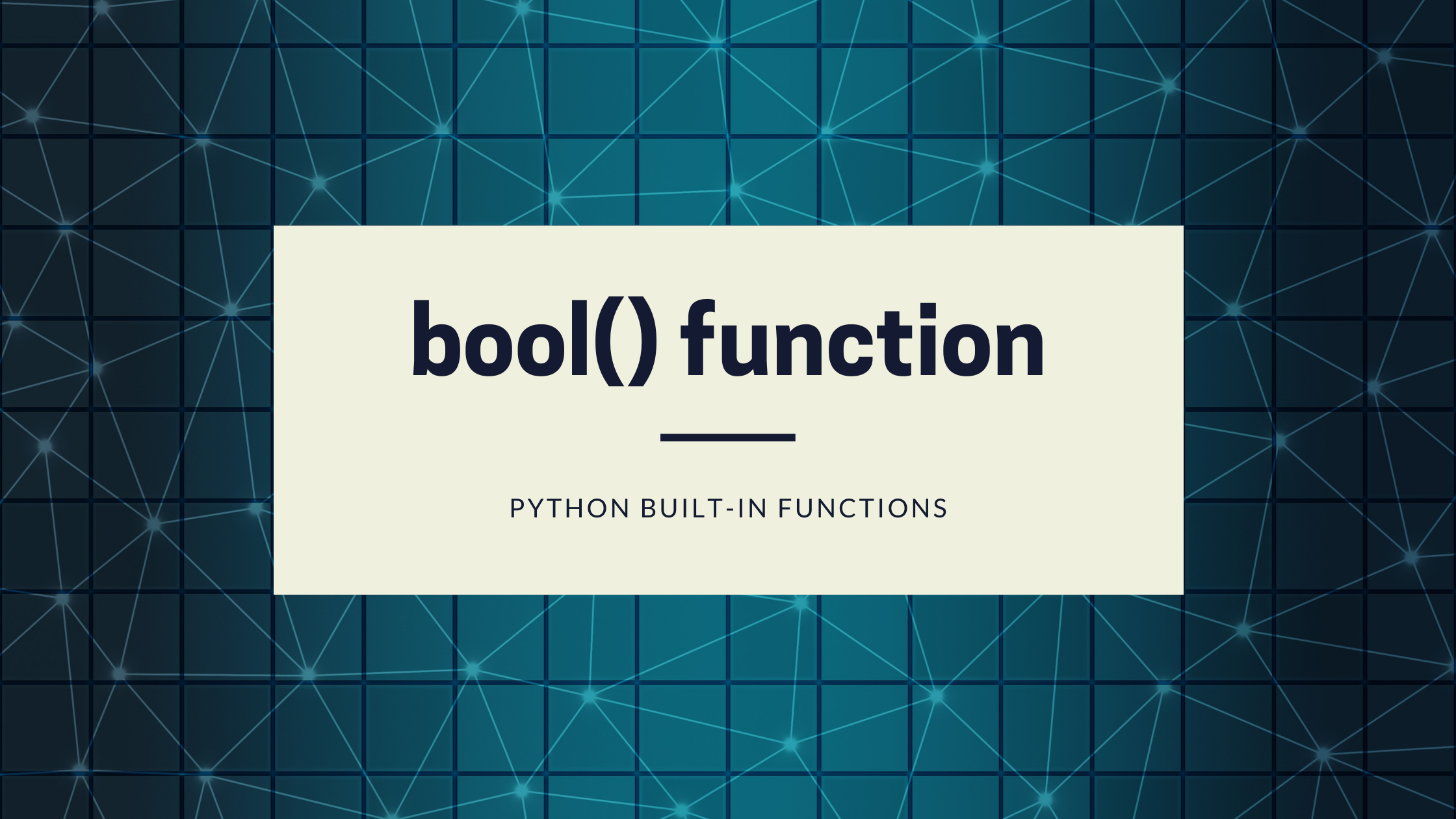# Ashutosh Writes# bool() function in Python

## Introduction

The `bool()` function converts a value to Boolean (`True` or `False`). If no value is passed to it, it returns `False`.

The syntax of `bool()` function is:

``````bool([value])
``````

where value can be integers, sequence, mapping, etc.

## Return Values

`bool()` function returns `True` if value is true, whereas `False` if value is false or no value is passed.

The following values are considered `False` in Python:

• `None`
• `False`
• Zero of any numeric type. For example, `0`, `0.0`, `0j`
• Empty sequence. For example, `()`, `[]`, `''`.
• Empty mapping. For example, `{}`
• objects of Classes that has `__bool__()` or `__len()__` method which returns `0` or `False`

All other values except these values are considered `True`.

## Example

``````value = [] # Empty List
print(bool(value))

value =  # List with one element
print(bool(value))

value = 0.0 # Floating point number 0
print(bool(value))

value = None # None
print(bool(value))

value = True # Boolean True
print(bool(value))

value = 'non-empty string' # Non-Empty String
print(bool(value))

value = ''
print(bool(value)) # Empty String
``````

Output:

``````False
True
False
False
True
True
False
``````

## Conclusion

In this part, we learned about the Python `bool()` function with the help of examples.